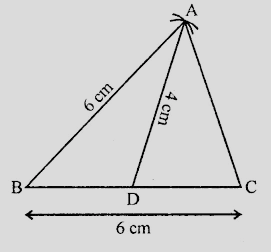# Construct a triangle $ABC$ such that $BC = 6\ cm, AB = 6\ cm$ and median $AD = 4\ cm$.

Given:

A triangle $ABC$ in which $BC = 6\ cm, AB = 6\ cm$ and median $AD = 4\ cm$.

To do:

We have to construct the given triangle.

Solution:Steps of construction:

(i) Draw a line segment $BC = 6\ cm$ and bisect it at $D$.

(ii) With centre $B$ and radius $6\ cm$ and with centre $D$ and radius $4\ cm$, draw arcs intersecting each other at $A$.

(iii) Join $AD, AB$ and $AC$.

Therefore,

$\triangle ABC$ is the required triangle.

Updated on: 10-Oct-2022

20 Views